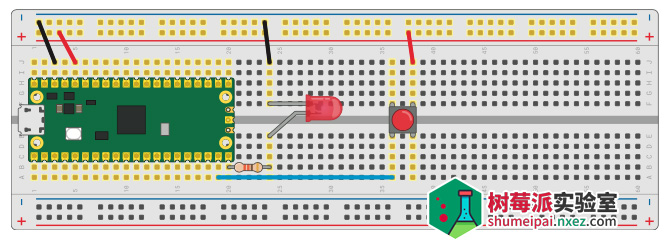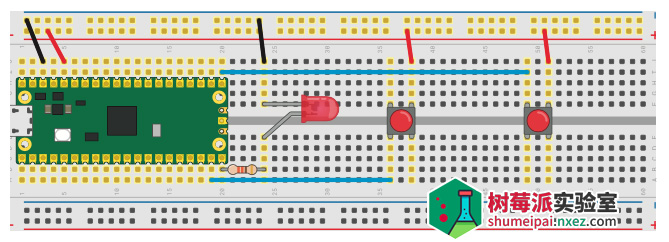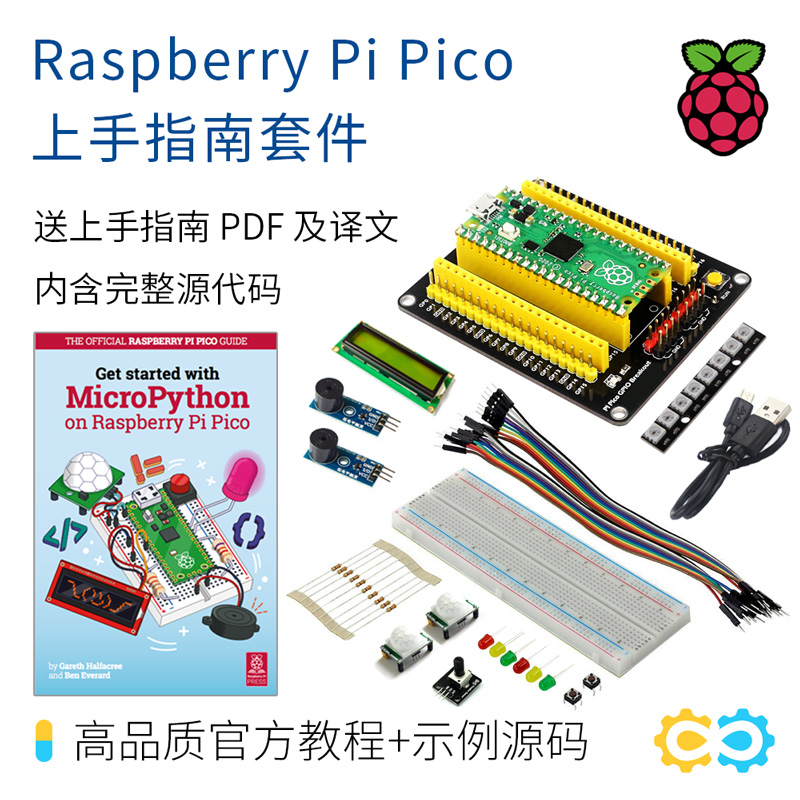# 用树莓派 Pico 编一个拼手速游戏– 树莓派 Pico
– 面包板
– 任何颜色的 LED 灯
– 一个 330Ω 电阻
– 两个按钮开关
– 若干公对公跳线
– 一根 microUSB 数据线

### 单人游戏Pico 上 GPIO 是有可编程电阻器的。在这本文的项目中，我们将它们设置为下拉电阻，这意味着当按钮按下时，引脚电压必须被拉高。

```import machine
import utime
```

```led = machine.Pin(15, machine.Pin.OUT)
button = machine.Pin(14, machine.Pin.IN, machine.Pin.PULL_DOWN)
```

MicroPython 中断请求以完全相同的方式工作，它允许某些东西（在这种情况下是按下按钮 开关）中断主程序。在某些方面，它和线程很相似，在主程序之外有一段代码。然而，与线程不 同的是，代码不是持续运行的，它只在中断被触发时运行。

```def button_handler(pin):
button.irq(handler=None)
print(pin)
```

```led.value(1)
utime.sleep(urandom.uniform(5, 10))
led.value(0)
```

```button.irq(trigger=machine.Pin.IRQ_RISING, handler=button_handler)
```

```button.irq(trigger=machine.Pin.IRQ_RISING | machine.Pin.IRQ_FALLING, andler=button_handler)
```

```import machine
import utime
import urandom

led = machine.Pin(15, machine.Pin.OUT)
button = machine.Pin(14, machine.Pin.IN, machine.Pin.PULL_DOWN)

def button_handler(pin):
button.irq(handler=None)
print(pin)

led.value(1)
utime.sleep(urandom.uniform(5, 10))
led.value(0)
button.irq(trigger=machine.Pin.IRQ_RISING, handler=button_handler)
```

```timer_start = utime.ticks_ms()
```

```timer_reaction = utime.ticks_diff(utime.ticks_ms(), timer_start)
print("Your reaction time was " + str(timer_reaction) + "milliseconds!")
```

```import machine
import utime
import urandom

led = machine.Pin(15, machine.Pin.OUT)
button = machine.Pin(14, machine.Pin.IN, machine.Pin.PULL_DOWN)

def button_handler(pin):
button.irq(handler=None)
timer_reaction = utime.ticks_diff(utime.ticks_ms(), timer_start)
print("Your reaction time was " + str(timer_reaction) + " milliseconds!")

led.value(1)
utime.sleep(urandom.uniform(5, 10))
led.value(0)
timer_start = utime.ticks_ms() button.irq(trigger=machine.Pin.IRQ_RISING, handler=button_handler)
```

### 双人游戏```right_button = machine.Pin(16, machine.Pin.IN, machine.Pin.PULL_DOWN)
```

```left_button = machine.Pin(14, machine.Pin.IN, machine.Pin.PULL_DOWN)
```

```button.irq(handler=None)
```

```left_button.irq(handler=None)
```

```right_button.irq(handler=None)
```

```left_button.irq(trigger=machine.Pin.IRQ_RISING, handler=button_handler)
```

```right_button.irq(trigger=machine.Pin.IRQ_RISING, handler=button_handler)
```

```import machine
import utime
import urandom

led = machine.Pin(15, machine.Pin.OUT)
left_button = machine.Pin(14, machine.Pin.IN, machine.Pin.PULL_DOWN)right_button = machine.Pin(16, machine.Pin.IN, machine.Pin.PULL_DOWN)

def button_handler(pin):
left_button.irq(handler=None)
right_button.irq(handler=None)
timer_reaction = utime.ticks_diff(utime.ticks_ms(), timer_start) print("Your reaction time was " + str(timer_reaction) +
" milliseconds!")

led.value(1)
utime.sleep(urandom.uniform(5, 10))
led.value(0)
right_button.irq(trigger=machine.Pin.IRQ_RISING, handler=button_handler)
left_button.irq(trigger=machine.Pin.IRQ_RISING, handler=button_handler)
```

#### 中断和中断处理函数

```fastest_button = None
```

```global fastest_button
fastest_button = pin
```

```while fastest_button is None:
utime.sleep(1)
```

```if fastest_button is left_button:
print("Left Player wins!")
elif fastest_button is right_button:
print("Right Player wins!")
```

```import machine
import utime
import urandom

led = machine.Pin(15, machine.Pin.OUT)
left_button = machine.Pin(14, machine.Pin.IN, machine.Pin.PULL_DOWN) right_button = machine.Pin(16, machine.Pin.IN, machine.Pin.PULL_DOWN)

fastest_button = None

def button_handler(pin):
left_button.irq(handler=None)
right_button.irq(handler=None)
global fastest_button
fastest_button = pin

led.value(1)
utime.sleep(urandom.uniform(5, 10))
led.value(0)
left_button.irq(trigger=machine.Pin.IRQ_RISING, handler=button_handler)
right_button.irq(trigger=machine.Pin.IRQ_RISING, handler=button_handler)

while fastest_button is None:
utime.sleep(1)

if fastest_button is left_button:
print("Left Player wins!")
elif fastest_button is right_button:
print("Right Player wins!")
```